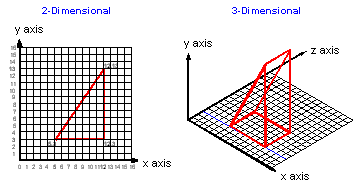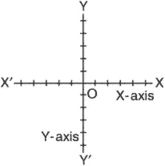# y-axis

(redirected from y-axes)
Also found in: Dictionary, Thesaurus, Medical.

## X-Y matrix

A group of rows and columns. The x-axis is the horizontal row, and the y-axis is the vertical column. An x-y matrix is the reference framework for two-dimensional structures, such as mathematical tables, display screens, digitizer tablets, dot matrix printers and 2D graphics images.Copyright © 1981-2019 by The Computer Language Company Inc. All Rights reserved. THIS DEFINITION IS FOR PERSONAL USE ONLY. All other reproduction is strictly prohibited without permission from the publisher.

## y-axisi. The lateral axis of the aircraft about which an aircraft pitches. Also called the pitch axis, normal axis, z-axis, and yawing axis.
ii. A vertical two-dimensional system of rectangular coordinates. The reference line on which distances above and below (north or south) it are marked, especially on a map, chart, or graph.
References in periodicals archive ?
Devices with Mirror Symmetries to x- and y-Axes and Contacts Not Lying on the x-and y-Axes, at Zero Shear Stress [[sigma].sub.xy] = 0
The 1st eigenfrequency of the RWA with the isolation system is observed in a low-frequency range at around 20 Hz in the x- and y-axes, which is the same as the results obtained from the launch vibration tests.
Considering the experiments of X- and Y-axes are independent, their errors are independent, too.
In order to obtain the complex scattered field at the object plane at a distance of z = d from the measurement plane, z = 0, back-propagation is applied following the definition of the wave propagation vector in the propagation axis (z-axis), [k.sub.z], as a function of wave propagation vectors in the x- and y-axes, [k.sub.x] and [k.sub.y], as follows
Its plane is asymmetric about the x- or/and y-axes. Each floor diaphragm is rigid in its own plane and has three degrees of freedom defined at the center of mass (CM).
The [E.sub.z] fields propagating along the x- and y-axes in the TE mode are replaced by the [E.sub.x] and [E.sub.y] fields, respectively, in the TM mode.
To present the entire reflector, the x- and y-axes must first be drawn during component design to establish a cross-shaped framework.
In this AMB system, the coil on the x- and y-axes circulates the same bias current ([i.sub.b]).
The X- and Y-axes run with 3150" min and accelerate up to 12.0 m/[s.sup.2], or 1.22g.
Be sure to give your graph a descriptive title and label the x- and y-axes.
This is due to the fact that there is no propagation of necking or that chain molecules orientate to almost the same degree in both X- and Y-axes. It should be noted here that some dark areas appeared after they were previously colored.

Site: Follow: Share:
Open / Close